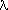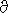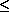# Planck function

The Planck function, B(T), is given by
B(T) = (13)/[exp(2/T) - 1]
where,= wavenumber in cm-1 = 104/,in µm.
T = temperature in degrees Kelvin1 = 2 h c2 = 1.191066 · 10-5 for radiance in units of mW · m-2 · steradian-1/cm-1.2 = h c / k = 1.438833 K cm
h is Planck's constant (6.62620 · 10-34 Joule second)
c is the speed of light (2.99793 · 108 m/second)
k is Boltzmann's constant (1.38062 · 10-23 Joule/K)is the Stefan-Boltzmann constant = 5.67 · 10-5 erg · s-1 · cm-2 · K-4 or mW · m-2 · K-4

Radiant Energy = A ·· T4

The brightness temperature, Tb, of a given radiance, R, is found with the inverse of the Planck function.

TbB-1(R) = (2 ·)/ loge [ 1 + (13/R) ]

The derivative of the Planck function is given byB/T = (124/T2) · exp(2/T)/[exp(2/T) - 1]2

Infrared approximation: for600 cm-1 and T300 K.

B(T)13 exp(-2/T)   mW · m-2 · steradian-1/cm-1
Tb(-2 ·)/ loge [ (13/R) ]B/T(124)/T2 · exp[-2/T]

Microwave Rayleigh Jeans approximation:(mm) = 300/f,= f/30 cm-1, f is in GHz

Wavenumbers,, are frequency units and are assumed to be in vacuum while wavelength is the wavelength specified within the medium. Typically, wavelengths are expressed as wavelength in air,a or wavelength in vacuum,v.10000/vv = na ·a= 10000/(na ·a)     na = 1.00027

 f cm-1 µm mm 0.66 GHz 0.02 454545 454.55 P band (SAR) 1.25 GHz 0.04 240000 240.00 C band (SAR) 5.33 GHz 0.18 56285 56.29 L band (SAR) 6.6 GHz 0.22 45454 45.45 MIMR (surface) 23 GHz 0.77 13043 13.04 AMSU-A 50 GHz 1.67 6000 6.00 AMSU-A 60 GHz 2.00 5000 5.00 AMSU-A 89 GHz 2.97 3371 3.37 AMSU-C 118 GHz 3.93 2542 2.54 MHS-X 183 GHz 6.10 1639 1.64 AMSU-B

B1/(2 · c2 · 10-18) · f2 · T = 9.2105 · 10-9 · f2 · T   mW · m-2 · steradian-1 ·/cm-1
Tb1.0857 · 108 R/f2B/T2 · c · k ·2 =1/2 ·2 =1/(2 · c2 · 10-18) · f2

 wavelengthmm frequencyGHz B(T=300K)mW/m2/ster/cm-1 dB/dTmW/m2/ster/cm-1/K (1/B) · dB/dT%/K 6.0 50 0.007 0.000023 0.335 3.0 100 0.027 0.000092 0.336 2.0 150 0.061 0.000207 0.337 1.5 200 0.109 0.000368 0.338

 wavelengthµm wavenumbercm-1 B(T=300K)mW/m2/ster/cm-1 dB/dTmW/m2/ster/cm-1/K (1/B) · dB/dT%/K 16.7 600 153.38 1.559 1.0 9.1 1100 81.49 1.441 1.8 6.2 1600 22.69 0.581 2.6 4.3 2300 2.35 0.086 3.7 3.7 2700 0.56 0.024 4.3 3.3 3000 0.18 0.009 4.8